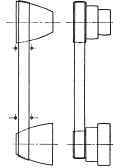It is often necessary to provide a range of speed variation in a shaft belted from a line shaft running at a constant rate. A familiar case of this kind is the ordinary lathe spindle. This may be done by shifting the belt from end to end of either a pair of cones or conoids, Fig. 106, depending on whether the belt is crossed or open. To work satisfactorily, a shipper must be located at each cone or conoid to guide the belt; otherwise it may climb. As these shippers give trouble and wear the belt, and the belt itself is unequally stretched, it is usual to approximate the conoids by stepped cones, or cone pulleys. In order to have the belt equally tight for each pair of steps, the diameters of the steps must be so proportioned that the length of belt remains constant.

It can be shown, geometrically, that for a crossed belt this condition is obtained when the sum of the diameters of each pair of steps is constant. In Fig. 106, a three-step cone with crossed belt is shown; and,adding together the diameters of the pair of steps connected by the belt, we have 11+6 = 17 for the constant of this cone pulley.

For an open belt, an extended calculation is necessary for diameters giving a constant belt length, and a simple graphic method for laying out the cones has been published by Mr. C. A. Smith in "Transactions of the American Society of Mechanical Engineers" (Vol. 10, p. 269). Here the distance between shafts and diameters for one cone pulley is assumed, or is known from the conditions of the drive.Fig. 105. Belt Drive with Main Pulleys of Different Diameters.

In Fig. 107, lay off A B equal to the distance between centers of shafts; and with these points as centers, draw circles C and D equal, respectively, to the maximum and minimum diameters of the given cone pulleys. Draw the belt line EF. From a point G, halfway between A and B, erect GH perpendicular to AB, and make it equal to 3.1416 AB (for the sake of space, it is not drawn to this scale in the cut). With 77 as a center, draw a circle tangent to EF; then the belt line of any other pair of pulleys must be tangent to this latter circle.Fig. 106. Cone Pulleys for Spindle and Cauntershaft.

Assume Dl the diameter of one of the pulleys, and draw a common tangent to circles D, and H, producing it past the center B. From B draw a perpendicular BF1 to the common tangent; and with BF1 as a radius, draw in the circle C1,which will give the pulley required to work with D1 and having approximately the same length of belt as on pulleys D and C. Continue the process until the required number of steps have been obtained. It must be noted that the limit for which this construction can be used is reached when the belt angle K is equal to 18˚. When the angle K is between 18° and 30°, proceed as follows:

Locate another point J on the line GH so that the distance HJ is equal to .298AB; draw a tangent to circle H, making an angle of 18° with the line of centers AB, and from point J draw an arc tangent to this tangent. Make all belt lines which are greater than 18° tangent to this arc.